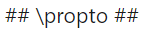# Angular deceleration of the Earth

Gold Member
how to do the problem then? I am struggling to understand
Is the overall method in post #31 not making sense, or are you hung up on the fact we don’t exactly know ##t## in that equation(like I was)?

Last edited:
Gold Member
how to do the problem then? I am struggling to understand
I suggest starting by figuring what is the period T and angular velocity ##\omega## in the year 1900. These are based on the given data. Then do it for 1977 based on the change given in the period. Then use the relevant equation from your original post. This is the same as in post #31 but in words. Remember to use consistent units either rotations/revolutions or radians but don't mix them.

Also, it's good practice to solve everything symbolically and put in numbers at the end when you have a formula for ##\alpha##.

Last edited:
•erobz
Gold Member
@Zoubayr , any progress understanding this problem? Have our last hints helped at all?

Zoubayr
@Zoubayr , any progress understanding this problem? Have our last hints helped at all?
I am having a really hard time with the question

Gold Member
I am having a really hard time with the question
Well, there has to be something specific that you are hung up on. Try articulating that?

Homework Helper
Gold Member
2022 Award
Just find the change in angular velocity rad/s, and divide that by 77 years (in seconds)?

Gold Member
As @erobz and @malawi_glenn are saying, start with your relevant equation. I'm going to use small ##t## for the time which passes, 77 years, and big ##T## for the period of the earth and ##\Delta T## for its change.

$$\omega_{77} = \omega_{00} + \alpha t$$ solve symbolically for the ##\alpha##

Now compute the two angular velocities from the given information. They give you the change in period over 365 revolutions (which we can take as 1 year) during time ##t##. With ##\theta## as the total angle we can write the angular velocities since these are averages as;

$$\omega_{00} = \frac{ \theta}{ T }$$

$$\omega_{77} = \frac{ \theta}{T + \Delta T}$$ so we have;

$$\frac{ \theta}{T + \Delta T} - \frac{ \theta}{ T } = \alpha t$$ at this point solve for ##\alpha## and then put the numbers in with consistent units. Hint: multiply both side by ##T(T+ \Delta T)##.

•erobz
Homework Helper
Gold Member
2022 Award
Just divide both sides by t. Modern calculators can handle the LHS as it is written

Gold Member
Just divide both sides by t. Modern calculators can handle the LHS as it is written
True, but then the student misses the joy of seeing that ##\alpha ∝\frac{ \Delta T}{T^2}##.

•erobz and SammyS
Homework Helper
Gold Member
2022 Award
Its not a proportionality (exact)

Gold Member
Its not a proportionality (exact)
Proportionality does not imply other relationships are not involved. We can say Newton's Gravity is proportional to ##\frac{1}{r^2}## does not mean that's all to it. I made the point the student would not see the beauty of the relationships if they just plugged the numbers in the LHS of the equation. I left it for the student to complete and see.

Homework Helper
Gold Member
2022 Award
If it were exact I would have used the equal sign.
## \propto ## means proportional toGold Member
Homework Helper
Gold Member
2022 Award
I used the symbol table above.
If you use a symbol from the symbol table, it means something else?

Homework Helper
Gold Member
2022 Award
I do not think you understand what "proportional to" means then...

Gold Member
I do not think you understand what "proportional to" means then...
What do you think I think it means then? And why are you so concerned?

Homework Helper
Gold Member
2022 Award
What do you think I think it means then? And why are you so concerned?
if y is proportional to x, then y = kx for some constant k.
This is not the case here.

Mentor
This seems like a good time to close this thread. The OP has been given a lot of help and there's not much more that we can do.

Thank you all for contributing here.

Jedi